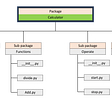# A walk-through Python Code Optimization:

“In this series I assume to explain a little bit of Python code optimization, beginning with a conceptual comprehension of how computers operate till simple Python benchmarks, to evaluate the running time of different constructors, methods, functions and so on. Going through Python memory storage and management.”

Something that has always got my attention is, how can computers make what they make? How can they display interfaces, images, colours and so forth? I read about it when I was younger and realize that the people who invented them were amazing, don’t you think?

Computers can process information thanks to their components, the hardware, but how can they read different information and display it? That’s a little tricky and complex, but we’ll try to simplify it further to get the main idea.

First of all, we shall explain how computers read or write, in other words, give or receive information. Computers can communicate and interact with other components, people and even with other PC, thanks to the binary notation. That is, a different way to express numbers, strings, images, words… If you aren’t familiar with that notation, I encourage you to keep reading.

To make an easier example we are going to start explaining the decimal notation, which we are so familiar with. And if you don’t recognize what decimal notation is, think about the numbers we use daily.

The decimal numbers as we know them, are representations of numbers in base 10. That means that we can only use a range of ten numbers to represent the number that we want, and we have to consider 0 also as a number, don’t forget him… so the range, as you see above is from o to 9. A sequence of numbers in base 10 can represent bigger numbers than 9. Let’s put an example to visualize what we are talking about.

If we only had 1 “slot” to place a number in base 10, we could only represent exactly one number from the range that we’ve seen a few moments earlier. In a mathematical notation it would be:

Where the “strange e” means inside, and the square brackets represent a range from 0 to 9.

If you’d like to represent a simple number, it would be easy, but if we’d like to represent a bigger number than 9, what should we do? Add an extra “slot” following the same rules as we only had one, in this way we could represent numbers until 99. And what are exactly is the right and left number from the 99?

Each “slot” corresponds to the number it’s storing itself, times 10 to the power of n, being “n” the slot number. The reason that we are multiplying it to 10^n it is because we are working in base 10. It seems complicated if we saw it in the more conceptual way, but it isn’t. Take a look:

Under the lines, (what we’ve called slots) we can find the order where we should read them and the value of “n” and above the X that has the same value that we saw previously. Basically, we work with them from right to left, and if you think on how you write numbers it makes plenty of sense. If you are writing numbers, at the moment that you reach 9, this one it transforms into a 0 and you add a 1 to the left.

Following the numeration, and powering it to base ten, we’ll determinate the unites of the number: tens, hundreds, one thousands, ten thousand, hundreds thousand…

The below photo has under the lines 10 to the “n” power, and the equivalence of what those powers represent.

So, if we’d like to say the number five thousand four hundred and twenty-three (5423), we should represent it as:

In a more mathematical way, we can write it:

Okay, up to now everything makes sense, but I haven’t told you anything new, what does that have to do with computers?

As we’ve mentioned, computers use binary notation, which is the same as decimal notation with the main difference that instead of base 10 — as you may be figuring — is in base 2. You may be guessing if base 10 implies a range [0 ,9], base 2 implies a range … [0, 1]. From here comes the name: Binary.

Before we touch base with how to rewrite base 10 numbers into base 2, or other bases, let’s put a similar same example as in base 10 but with a binary notation to know how those numbers are represented.

As in the decimal notation, we are going to have “slots” and we will read them in the same order, also the numeration of the “slots” are going to be the same. The difference is going to be the base of the exponent part, where instead of 10, it’s going to be 2. Look below:

Once we have the “equivalence” for the binary representation, we can try to represent a binary number and rewrite it in a decimal notation.

E.g., 1

E.g.,2

Now that we know how to read binary notation, we would have to know how to rewrite numbers in other bases to base 2, and to fully understand that, we have to go back to divisions, where we’re going to get in touch with two main concepts: DIV and MOD.

In a normal division we can find 4 parts: Divisor, Dividend, Quotient and Remainder.

We can express all divisions as follow:

In the below image you’ll find:

· Dividend = 13.

· Divisor = 5.

· Quotient = 2.

· Remainder = 3.

All rational numbers who have a finite number of decimals, have a fraction representation, they can be expressed as Equation 1.

For convention we’ll change the names for operators. The quotient and reminder can be expressed by, in this particular example:

The double slash ( // ) and the percentage ( % ) symbols, are the mathematic operators for DIV and MOD respectively. If we substitute the names (left side of the above equation) by their mathematic form (right side), we can express the Dividend as:

To conclude, we only have to change our specific values for dividend and divisor for generic notation like a and b.

In the next episode, Episode 2: Base transformation, we’ll see how we can rewrite numbers in base 10 and others to base 2, how we should write an algorithm to implement that, and what are actually the slots. I hope you’ve understood everything we’ve explained and feel free to ask if you have some doubts.

--

--

--

## More from Alex Abades Grimes

“If you can’t explain it simply, you don’t understand it well enough” -Albert Einstein.

Love podcasts or audiobooks? Learn on the go with our new app.

## Day 29 of 100 Days of VR: Spawning New Enemies in Unity and Dealing With “Ghost” Colliders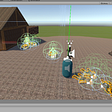## What is the simplest way to test my local Laravel apps on my mobile?## Decoding the language of great software products: A tech dictionary for businesses## Deploying Django to AWS using a VPC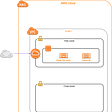## Edge Application: Streaming Multiple Video Sources with Gstreamer and Ceph Object Storage Part 2/3## Algorithm Talk! Day 1: Bucket Sort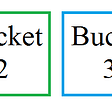## Connecting to Amazon Redshift with TeamSQL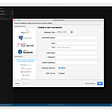“If you can’t explain it simply, you don’t understand it well enough” -Albert Einstein.

## CRUNCH COUNTER IN PYTHON## How to Dual Boot Windows 10 along with Windows 11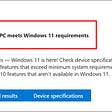## Python script installed which is not on PATH.## What are python packages and how to fix a python packages error “TypeError: ‘module’ object is not…